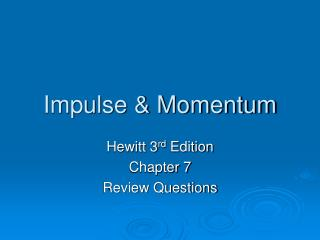DownloadDownload PresentationImpulse & Momentum

# Impulse & Momentum

Télécharger la présentation## Impulse & Momentum

- - - - - - - - - - - - - - - - - - - - - - - - - - - E N D - - - - - - - - - - - - - - - - - - - - - - - - - - -
##### Presentation Transcript

1. Impulse & Momentum Hewitt 3rd Edition Chapter 7 Review Questions

2. Distinguish between mass and momentum. (7.1)Which is inertia?Which is inertia in motion? • Mass is the amount of matter in an object. • Momentum is the quantity of motion. • Mass is inertia • Momentum is inertia in motion.

3. 2.a. Which has the greater mass, a heavy truck at rest or a rolling skateboard? (7.1) • The truck. • b. Which has greater momentum? • The rolling skate board.

4. 3. Distinguish between impact and impulse. (7.2) • Impact refers to a force and is measured in Newtons. • Impulse is impact force x time and is measured in Newton-seconds. • Which designates a force? • Impact designates a force. • Which is force multiplied by time? • Impulse = Force x Time

5. 4. When the force of impact on an object is extended in time, does the impulse increase or decrease? (7.2) • Increases • Follow through

6. 5. Distinguish between impulse and momentum. (7.2) • Impulse is force x time. • Momentum is inertia in motion. • Momentum is mass x velocity. • “Impulse is the thing that you DO, momentum is the thing that you SEE.”

7. 6. Does impulse equal momentum, or a change in momentum? (7.2) • Change in momentum.

8. For a constant force, suppose the duration of impact on an object is doubled. (7.2) a. How much is the impulse increased? • It is doubled. • b. How much is the resulting change in momentum increased? • It is doubled.

9. 8. In a car crash, why is it advantageous for an occupant to extend the time during which when the collision takes place? (7.2) • Greater time means less force.

10. 9. If the time of impact in a collision is extended by four times, how much does the force of impact change? (7.2) • It is reduced to one-fourth.

11. 10. Why is it advantageous for a boxer to ride with the punch? (7.2) • Greater time means less force • Why should he avoid moving into an oncoming punch? • Less time means greater force

12. 11. Visualize yourself on a skateboard. (7.3) • a. When you throw a ball, do you experience an impulse? • Yes • b. Do you experience an impulse when you catch a ball of the same speed? • Yes • c. Do you experience an impulse when you catch it and then throw it out again? • Yes • d. Which impulse is greatest? • Catching and throwing, the impulse is greatest.

13. 12. Why is more impulse delivered during a collision when bouncing occurs than during one when it doesn’t (7.3) • The change in momentum is greater so the impulse is greater.

14. 13. Why is the Pelton Wheel an improvement over paddle wheels with flat blades? (7.3) • It causes a greater change in the fluid’s momentum and so provides more impulse.

15. 14. In terms of momentum conservation, why does a cannon recoil when fired? (7.4) • The cannon’s momentum must be equal and opposite to that of the cannonball.

16. 15. What does it mean to say that momentum is conserved? (7.4) • The momentum before the collision is the same as the momentum after the collision.

17. 16. Distinguish between an elastic and an inelastic collision. (7.5) • An elastic collision involves bounces. Objects become distorted and generate heat during an inelastic collision.

18. 17. Imagine that you are hovering next to the space shuttle in an earth-orbit and your buddy of equal mass who is moving at 4 km/h with respect to the ship bumps into you. If he holds onto you, how fast do you both move with respect to the ship? (7.5) • The mass doubles so the speed is halved • 2 km/h

19. 18. Is momentum conserved for colliding objects that are moving at angles to one another? Explain (7.6) • Yes. Resulting motions follow the vector rules.